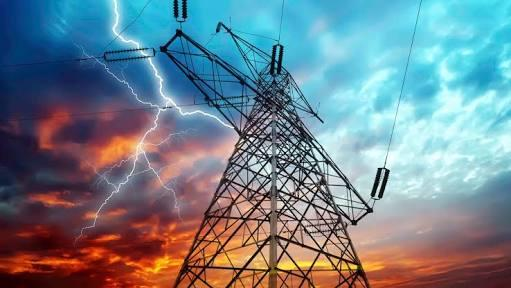# What Do You Know About Electricity?

10 Questions | Total Attempts: 12SettingsAccording to Microsoft Encarta Dictionary, electricity is a fundamental form of kinetic or potential energy created by the free or controlled movement of charged particles such as electrons, positrons, and ions.

Related Topics
• 1.
One of the following is an earlier researcher on the subject electricity:
• A.

Emmanuel

• B.

Thales

• C.

Aristotle

• D.

Ferdinard

• 2.
Which of the animal possess tangible electric current?
• A.

Man

• B.

Crab

• C.

Eel

• D.

Snake

• 3.
What is the S.I. unit for Electric current?
• A.

Watts

• B.

Voltage

• C.

Ohms

• D.

Ampere

• 4.
What is the S.I. unit for electric power?
• A.

Watt

• B.

Voltage

• C.

Joules

• D.

Kinet

• 5.
Who is the father of the modern study of electricity?
• A.

Kem Dale

• B.

Steve Jobs

• C.

Robert Noyce

• D.

• 6.
What type of electricity did the first Thomas Edison light bulb used?
• A.

Alternating Current

• B.

Ultra alternating current

• C.

Direct Current

• D.

Dwindling Current

• 7.
Who is credited with the invention of power transmission system?
• A.

Mike Spencer

• B.

Andrew Carnegie

• C.

Thomas Edison

• D.

Nikola Telsa

• 8.
Alessandro Volta is known for one of the following invention
• A.

Voltaic Pile

• B.

Car battery

• C.

Van da graff generator

• D.

Lithium ion battery

• 9.
What is the unit for measuring electric potential?
• A.

Pounds

• B.

Voltage

• C.

Wattage

• D.

Mileage

• 10.
Which of the following laws explains electromagnetism?
• A.

Viktor's theorem

• B.

Ohm's law

• C.

Ampere's law

• D.

Archimedes Principle